Select Page

# General Network Concepts

General Network Concepts

Contents

Define Electric Charge 2

Describe basic characteristics and properties of Electric Charge 2

Define basic Network Elements with their Units and Symbols 3

Describe behaviour, different Laws, properties, and combinations of Resistor(R) 3

Define Ohm’s Law 4

Describe behaviour, different properties, and combinations of Inductor(L) 4

Describe Behaviour, different properties, and combinations of Capacitor(C) 5

Define Short and Open Circuit 6

Define Various Electrical Quantities 6

Describe Joules’ Law of Heating 7

Define Thermal Efficiency 7

Define Active & Passive, Linear & Non-linear, and Unilateral & Bilateral Components 8

Differentiate Active & Passive, Linear & Non-linear, and Unilateral & Bilateral Components 8

Define Lumped and Distributed parameters 9

Define Voltage and Current Sources 9

Classify Voltage and Current Sources 9

Apply Source transformation to convert Voltage source to Current source and vice-versa 10

Describe the methods of solving the Networks 10

Describe Kirchhoff’s Current Law (KCL) 11

Explain Current-Division Rule 14

Describe Kirchhoff’s Voltage Law (KVL) 15

Explain Voltage Division Rule 15

Conversion of Star-to-Delta and Delta-to-Star 15

Apply Star-Delta conversion to simplify the circuits 21

Describe Nodal Method or Analysis in a Network 22

Describe Mesh Method or Analysis in a Network 22

Describe the concept of Super-Node and Super-Mesh Analysis 23

# Define Electric Charge

Electric charge is a fundamental property of matter that results in an attraction or repulsion between objects. It is a physical property of matter that describes the amount of electrical energy contained within a given particle or system. Electric charge is quantized, meaning that it exists in discrete units, known as elementary charges, which are carried by subatomic particles such as protons and electrons. The SI unit of electric charge is the Coulomb (C).

# Describe basic characteristics and properties of Electric Charge

Electric charge has two fundamental properties: magnitude and polarity. The magnitude of an electric charge is given by the amount of charge on a particle and is measured in Coulombs. The polarity of an electric charge can be positive or negative and determines the direction of the electric field around the charge.

Electric charges can be either stationary or in motion. When an electric charge is in motion, it produces a magnetic field as well as an electric field. Electric charges also interact with each other through the electric and magnetic fields they produce, resulting in attraction or repulsion between charges.

Electric charge is conserved, meaning that the total amount of electric charge in a system remains constant, even as charges are transferred from one object to another. Additionally, electric charge is quantized, meaning that it exists in discrete units, known as elementary charges, which are carried by subatomic particles such as protons and electrons.

# Define basic Network Elements with their Units and Symbols

A network element is a basic component of an electrical circuit that performs a specific function. The most commonly used network elements are resistors, capacitors, and inductors.

A resistor is a network element that resists the flow of electrical current and is measured in ohms (Ω). Its symbol is a rectangle with a zigzag line inside.

A capacitor is a network element that stores electrical energy and is measured in farads (F). Its symbol is two parallel lines with a gap in between.

An inductor is a network element that stores energy in a magnetic field and is measured in henries (H). Its symbol is a coil of wire.

# Describe behaviour, different Laws, properties, and combinations of Resistor(R)

Resistors are passive network elements that are used to control the flow of electrical current in a circuit. They exhibit the property of resistance, which is the opposition of a material to the flow of electric current.

The behavior of a resistor is described by Ohm’s law, which states that the current through a conductor between two points is directly proportional to the voltage across the two points. This relationship is expressed by the formula V=IR, where V is the voltage, I is the current, and R is the resistance.

Resistors can be connected in series or parallel to achieve different properties. When resistors are connected in series, their resistances add up. When they are connected in parallel, their reciprocals add up, and the total resistance is less than the smallest resistance in the network.

Resistors also have power ratings, which describe the amount of power that they can dissipate without being damaged. The power rating of a resistor is typically given in watts.

# Define Ohm’s Law

Ohm’s Law is a fundamental principle in electrical engineering and physics that relates the voltage, current, and resistance in an electrical circuit. It states that the current flowing through a conductor between two points is directly proportional to the voltage across the two points, provided the temperature and other physical conditions remain constant. Mathematically, Ohm’s Law is expressed as V=IR, where V is the voltage, I is the current, and R is the resistance. This law is named after Georg Simon Ohm, a German physicist who first formulated it in the early 19th century. Ohm’s Law is widely used in the design and analysis of electrical circuits, and is essential for understanding the behavior of network elements such as resistors.

# Describe behaviour, different properties, and combinations of Inductor(L)

An inductor is a network element that stores energy in a magnetic field. It is typically made of a coil of wire and is measured in henries (H). The behavior of an inductor is governed by Faraday’s law of electromagnetic induction, which states that an electric current is induced in a conductor when it is exposed to a changing magnetic field.

In a circuit, an inductor opposes changes in current flow by generating a voltage that is proportional to the rate of change of current. This property is called inductance and is denoted by L. The unit of inductance is the henry (H).

Inductors can be connected in series or parallel to achieve different properties. When inductors are connected in series, their inductances add up. When they are connected in parallel, their reciprocals add up, and the total inductance is greater than the largest inductance in the network.

Inductors also have a property called reactance, which is the opposition of an inductor to the flow of alternating current (AC). The reactance of an inductor is directly proportional to the frequency of the AC signal and the inductance of the inductor.

# Describe Behaviour, different properties, and combinations of Capacitor(C)

A capacitor is a network element that stores electrical energy in an electric field. It is typically made of two conductive plates separated by a dielectric material and is measured in farads (F). The behavior of a capacitor is governed by Coulomb’s law, which states that the electric charge on a conductor is proportional to the voltage applied to the conductor.

In a circuit, a capacitor resists changes in voltage by storing electrical charge. This property is called capacitance and is denoted by C. The unit of capacitance is the farad (F).

Capacitors can be connected in series or parallel to achieve different properties. When capacitors are connected in series, their reciprocals add up, and the total capacitance is less than the smallest capacitance in the network. When they are connected in parallel, their capacitances add up.

Capacitors also have a property called reactance, which is the opposition of a capacitor to the flow of alternating current (AC). The reactance of a capacitor is inversely proportional to the frequency of the AC signal and the capacitance of the capacitor.

# Define Short and Open Circuit

A short circuit is a connection between two points in an electrical circuit that has very low or zero resistance, causing a large current to flow through the circuit. This can result in damage to the circuit and pose a safety hazard. In a short circuit, the voltage across the two points is zero, and the current flowing through the circuit is limited only by the resistance of the conductors and the power supply.

An open circuit is a break in an electrical circuit that prevents current from flowing through it. This can be caused by a faulty component or a disconnected wire. In an open circuit, the voltage across the break is equal to the voltage of the power supply, and no current flows through the circuit.

# Define Various Electrical Quantities

There are several electrical quantities that are commonly used in electrical circuits. These quantities are:

1. Voltage: Voltage, also known as electric potential difference, is the measure of the difference in electric potential between two points in a circuit. It is measured in volts (V) and is represented by the symbol V.
2. Current: Electric current is the flow of electric charge through a conductor. It is measured in amperes (A) and is represented by the symbol I.
3. Resistance: Resistance is the measure of the opposition to the flow of electric current through a conductor. It is measured in ohms (Ω) and is represented by the symbol R.
4. Power: Power is the rate at which energy is transferred or converted per unit time. In electrical circuits, power is often measured in watts (W) and is represented by the symbol P.
5. Energy: Electrical energy is the energy that is associated with electric charges due to their position or motion. It is measured in joules (J).
6. Frequency: Frequency is the number of cycles of a periodic waveform that occur in a second. It is measured in hertz (Hz) and is represented by the symbol f.
7. Impedance: Impedance is the total opposition to the flow of electric current in an alternating current (AC) circuit. It is a combination of resistance, capacitance, and inductance and is measured in ohms (Ω).
8. Reactance: Reactance is the measure of the opposition to the flow of electric current due to capacitance or inductance. It is measured in ohms (Ω) and is represented by the symbols Xc and Xl respectively for capacitive and inductive reactance.
9. Conductance: Conductance is the measure of the ease with which electric current flows through a material. It is the reciprocal of resistance and is measured in Siemens (S).
10. Admittance: Admittance is the measure of the ease with which electric current flows through a circuit. It is the reciprocal of impedance and is measured in Siemens (S).

# Describe Joules’ Law of Heating

Joule’s Law of Heating is a physical law that describes the relationship between the heat produced by an electrical conductor and the current flowing through it. It states that the heat generated in a conductor is proportional to the square of the current flowing through it, the resistance of the conductor, and the time for which the current flows. The law is expressed as H = I2RT, where H is the heat generated in joules, I is the current in amperes, R is the resistance in ohms, and T is the time in seconds. This law has a number of practical applications, such as in the design of electrical heaters, in determining the energy dissipated in electrical circuits, and in the measurement of power consumption.

# Define Thermal Efficiency

Thermal efficiency is a measure of the efficiency with which a thermal system converts fuel or energy input into useful output, such as electricity or heat. It is defined as the ratio of the output energy or work produced by the system to the input energy or fuel supplied to the system, expressed as a percentage. For example, the thermal efficiency of a power plant is the ratio of the electricity generated to the fuel input, while the thermal efficiency of a furnace is the ratio of the heat output to the fuel input. The higher the thermal efficiency, the more efficient the system is at converting input energy into useful output.

# Define Active & Passive, Linear & Non-linear, and Unilateral & Bilateral Components

Components in an electrical circuit can be classified as active or passive, linear or non-linear, and unilateral or bilateral. Active components are those that can provide energy to a circuit, such as voltage or current sources, while passive components are those that cannot, such as resistors, capacitors, and inductors. Linear components are those whose electrical properties do not change with changes in voltage or current, while non-linear components do. Unilateral components are those that allow current to flow in only one direction, such as diodes, while bilateral components allow current to flow in both directions, such as resistors. Understanding these classifications is important for designing and analyzing electrical circuits.

# Differentiate Active & Passive, Linear & Non-linear, and Unilateral & Bilateral Components

Active components are those that can add energy to a circuit or system, such as a battery or an amplifier. Passive components are those that do not add energy but can store or dissipate energy, such as resistors, capacitors, and inductors.

Linear components are those that have a linear relationship between their inputs and outputs, meaning that their behavior can be described by a straight line on a graph. Examples include resistors, capacitors, and inductors. Nonlinear components, on the other hand, have a nonlinear relationship between their inputs and outputs and cannot be described by a straight line. Examples include diodes, transistors, and nonlinear resistors.

Unilateral components are those that allow current to flow in only one direction, such as diodes. Bilateral components are those that allow current to flow in both directions, such as resistors.

# Define Lumped and Distributed parameters

Lumped parameters refer to the characteristics of a system or circuit that can be represented as discrete values concentrated at specific points in the system. Examples include the resistance, capacitance, and inductance of a circuit.

Distributed parameters, on the other hand, refer to the characteristics of a system or circuit that vary continuously throughout the system. Examples include the propagation delay of a transmission line or the impedance of a distributed circuit element.

# Define Voltage and Current Sources

A voltage source is an electronic component that generates a fixed or variable voltage output, independent of the current drawn by the circuit. Examples include batteries, DC power supplies, and AC voltage sources.

A current source, on the other hand, is an electronic component that generates a fixed or variable current output, independent of the voltage across the circuit. Examples include transistors, operational amplifiers, and controlled current sources. Current sources are less common in practical circuits than voltage sources.

# Classify Voltage and Current Sources

In electrical circuits, voltage and current sources are the two basic types of sources. A voltage source is a component that provides a constant voltage to a circuit, regardless of the current flowing through it. It can be modeled as a series combination of an ideal voltage source and a series resistance. The ideal voltage source has zero internal resistance, meaning that it can supply a constant voltage regardless of the current. The series resistance represents the resistance of the wires or other components in the circuit that connect to the source.

On the other hand, a current source is a component that provides a constant current to a circuit, regardless of the voltage across it. It can be modeled as a parallel combination of an ideal current source and a shunt resistance. The ideal current source has infinite internal resistance, meaning that it can supply a constant current regardless of the voltage. The shunt resistance represents the resistance of the path that the current takes in the circuit.

# Apply Source transformation to convert Voltage source to Current source and vice-versa

Source transformation is a technique used to convert a voltage source to an equivalent current source, or vice versa. This technique can be useful in circuit analysis and design, as it can simplify calculations and allow for easier comparisons between different circuits.

To convert a voltage source to a current source, the source voltage is divided by the resistance connected across its terminals, and the resulting current is used as the equivalent current source. The equivalent shunt resistance is equal to the resistance across the terminals of the voltage source.

Conversely, to convert a current source to a voltage source, the source current is multiplied by the resistance connected in series with it, and the resulting voltage is used as the equivalent voltage source. The equivalent series resistance is equal to the resistance in series with the current source.

# Describe the methods of solving the Networks

There are several methods for solving electrical networks, which are collections of interconnected components such as resistors, capacitors, and inductors. These methods are used to determine the voltages and currents in the network for a given set of input values.

One method is nodal analysis, which involves applying Kirchhoff’s current law (KCL) at each node in the circuit. Nodes are points in the circuit where two or more components meet, and KCL states that the sum of the currents entering a node must equal the sum of the currents leaving the node. By applying KCL at each node and using Ohm’s law to relate the node voltages to the component currents, a system of linear equations can be obtained that can be solved to determine the node voltages.

Another method is mesh analysis, which involves applying Kirchhoff’s voltage law (KVL) around each loop in the circuit. Loops are closed paths in the circuit that do not contain any nodes, and KVL states that the sum of the voltage drops around a loop must equal the sum of the voltage rises. By applying KVL around each loop and using Ohm’s law to relate the loop currents to the component voltages, a system of linear equations can be obtained that can be solved to determine the loop currents.

Thevenin’s theorem is another method that involves finding the equivalent Thevenin circuit, which consists of a voltage source in series with a resistor, that represents the behavior of the original circuit as seen from a specific pair of terminals. The equivalent Thevenin circuit is obtained by calculating the open-circuit voltage across the terminals and the equivalent resistance seen from the terminals, which can be found by removing all sources and calculating the resistance between the terminals.

# Describe Kirchhoff’s Current Law (KCL)

Kirchhoff’s Current Law, often shortened to KCL, states that “The algebraic sum of all currents entering and exiting a node must equal zero.”

This law is used to describe how a charge enters and leaves a wire junction point or node on a wire.

Armed with this information, let’s now take a look at an example of the law in practice, why it’s important, and how it was derived.

Parallel Circuit Review

Let’s take a closer look at that last parallel example circuit: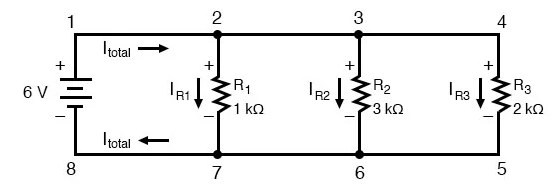Solving for all values of voltage and current in this circuit: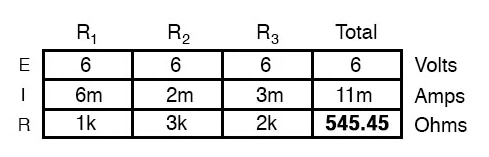At this point, we know the value of each branch current and of the total current in the circuit. We know that the total current in a parallel circuit must equal the sum of the branch currents, but there’s more going on in this circuit than just that. Taking a look at the currents at each wire junction point (node) in the circuit, we should be able to see something else: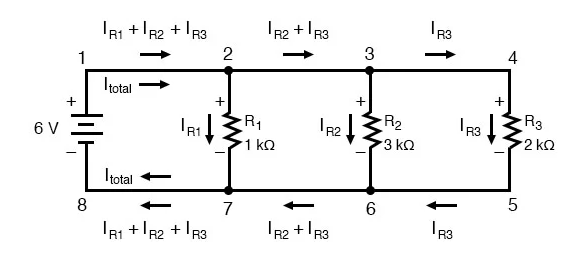Currents Entering and Exiting a Node

At each node on the positive “rail” (wire 1-2-3-4) we have current splitting off the main flow to each successive branch resistor. At each node on the negative “rail” (wire 8-7-6-5) we have current merging together to form the main flow from each successive branch resistor. This fact should be fairly obvious if you think of the water pipe circuit analogy with every branch node acting as a “tee” fitting, the water flow splitting or merging with the main piping as it travels from the output of the water pump toward the return reservoir or sump.

If we were to take a closer look at one particular “tee” node, such as node 6, we see that the current entering the node is equal in magnitude to the current exiting the node: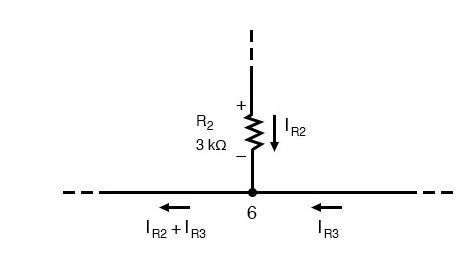From the top and from the right, we have two currents entering the wire connection labeled as node 6. To the left, we have a single current exiting the node equal in magnitude to the sum of the two currents entering. To refer to the plumbing analogy: so long as there are no leaks in the piping, what flow enters the fitting must also exit the fitting. This holds true for any node (“fitting”), no matter how many flows are entering or exiting. Mathematically, we can express this general relationship as such: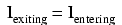Kirchhoff’s Current Law

Mr. Kirchhoff decided to express it in a slightly different form (though mathematically equivalent), calling it Kirchhoff’s Current Law (KCL):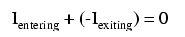Summarised in a phrase, Kirchhoff’s Current Law reads as such:

“The algebraic sum of all currents entering and exiting a node must equal zero”

That is, if we assign a mathematical sign (polarity) to each current, denoting whether they enter (+) or exit (-) a node, we can add them together to arrive at a total of zero, guaranteed.

Taking our example node (number 6), we can determine the magnitude of the current exiting from the left by setting up a KCL equation with that current as the unknown value: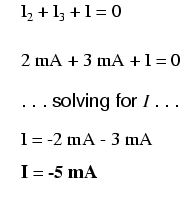The negative (-) sign on the value of 5 milliamps tells us that the current is exiting the node, as opposed to the 2 milliamp and 3 milliamp currents, which must both be positive (and therefore entering the node). Whether negative or positive denotes current entering or exiting is entirely arbitrary, so long as they are opposite signs for opposite directions and we stay consistent in our notation, KCL will work.

Together, Kirchhoff’s Voltage and Current Laws are a formidable pair of tools useful in analyzing electric circuits. Their usefulness will become all the more apparent in a later chapter (“Network Analysis”), but suffice it to say that these Laws deserve to be memorized by the electronics student every bit as much as Ohm’s Law.

# Explain Current-Division Rule

The Current-Division Rule is used to calculate the amount of current flowing through each branch of a parallel circuit. The rule states that the current flowing through each branch is proportional to the resistance of that branch. Mathematically, the rule can be expressed as:

I1 = (R2 / (R1 + R2)) x I

I2 = (R1 / (R1 + R2)) x I

Where I is the total current flowing through the parallel circuit, R1 and R2 are the resistances of the two branches, I1 and I2 are the currents flowing through each branch, respectively.

# Describe Kirchhoff’s Voltage Law (KVL)

Kirchhoff’s Voltage Law (KVL) states that the sum of voltages around any closed loop in a circuit is equal to zero. This law is based on the principle of conservation of energy, which states that energy cannot be created or destroyed, only conserved. KVL can be used to solve for the unknown voltages in a circuit by setting the sum of voltages around a closed loop equal to zero. This equation can be rearranged to solve for the unknown voltages.

# Explain Voltage Division Rule

The Voltage Division Rule is used to calculate the voltage across each resistor in a series circuit. The rule states that the voltage across each resistor is proportional to the resistance of that resistor. Mathematically, the rule can be expressed as:

V1 = (R1 / (R1 + R2)) x V

V2 = (R2 / (R1 + R2)) x V

Where V is the total voltage across the series circuit, R1 and R2 are the resistances of the two resistors, V1 and V2 are the voltages across each resistor, respectively.

# Conversion of Star-to-Delta and Delta-to-Star

The typical three-phase networks use two main methods by names which specify the way in which resistances are allied. In a star connection of the network, the circuit can be connected in symbol ‘∆’ model, similarly in a delta connection of the network; the circuit can be connected in symbol ‘∆’. We know that we can change the T-resistor circuit into the Y-type circuit for generating an equivalent Y- model network. Similarly, we can change the п-resistor circuit for generating an equivalent ∆- model network. So now it is very clear what is a star network circuit and delta network circuit, and how they transform into Y- model network as well as ∆- model network by using T-resistor and п-resistor circuits.

Star to Delta Conversion

In star to delta conversion, the T-resistor circuit can be transformed to Y-type circuit to generate an equivalent Y- model circuit. The star to delta conversion can be defined as the value of the resistor on any one side of the Delta network, and the addition of all the two resistor product combinations in the stat network circuit separate with the star resistor which is placed straightly opposite to the delta resistor being found. The star-delta transformation derivation is discussed below.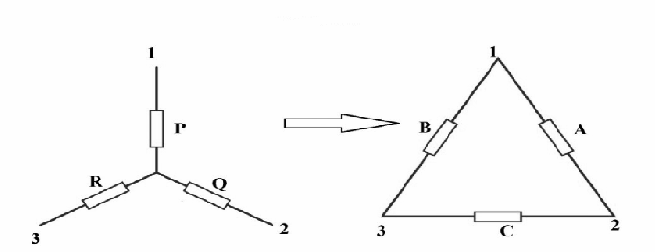For resistor A = XY + YZ + ZX/Z

For resistor B = XY + YZ + ZX/Y
For resistor C = XY + YZ + ZX/X
By separating out every equation with the denominator value we finish with 3-separate conversion formulas that can be utilized to change any Delta resistive circuit into an equivalent star circuit that is shown below.
For resistor A = XY + YZ + ZX/Z = XY/Z + YZ/Z + ZX/Z = (XY/Z) +Y+X
For resistor B = XY + YZ + ZX/Y = XY/Y + YZ/Y + ZX/Y= (ZX/Y) + X+Z

For resistor C = XY + YZ + ZX/X = XY/X + YZ/X + ZX/X= (YZ/X) +Z+Y
So, the final equations for the star to delta conversion are
A= (XY/Z) +Y+X, B= (ZX/Y) + X+Z, C= (YZ/X) +Z+Y

In this type of conversion, if the entire resistors values in the star connection are equal then the resistors in the delta network will be thrice of the star network resistors.

Resistors in Delta Network = 3 * Resistors in Star Network

For Example

The star-delta transformation problems are the best examples to understand the concept. The resistors in star network are denoted with X, Y, Z, and the values of these resistors are X= 80 ohms, Y= 120 ohms, and Z = 40 ohms, then the A and B and C values are followed.

A= (XY/Z) +Y+X

X= 80 ohms, Y= 120 ohms, and Z = 40 ohms

Substitute these values in the above formula

A = (80 X 120/40) + 120 + 80 = 240 + 120 + 80 = 440 ohms

B= (ZX/Y) + X+Z

Substitute these values in the above formula

B = (40X80/120) + 80 + 40 = 27 + 120 = 147 ohms

C= (YZ/X) +Z+Y

Substitute these values in the above formula

C = (120 x 40/80) + 40 + 120 = 60 + 160 = 220 ohms

Delta to Star Conversion

In delta to star conversion, the ∆-resistor circuit can be transformed to Y-type circuit to generate an equivalent Y- model circuit. For this, we require a conversion formula for comparing the different resistors to every other among the different terminals. The delta star transformation derivation is discussed below.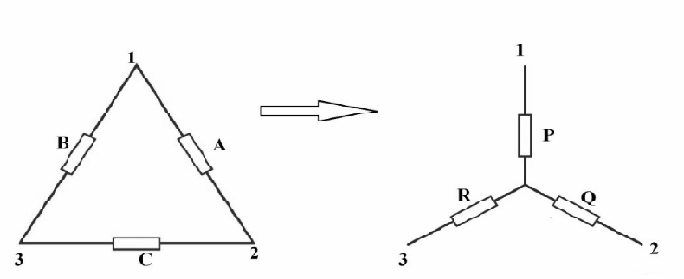Evaluate the resistances among the two terminals like 1 & 2.

X + Y = A in parallel with B +C

X + Y = A (B+C)/ A+B+C (Equation-1)

Evaluate the resistances among the two terminals like 2 & 3.

Y + Z = C in parallel with A + B

Y + Z = C (A + B)/ A + B + C (Equation-2)

Evaluate the resistances among the two terminals like 1 & 3.

X + Z = B in parallel with A + C

X + Z = B (A + C)/ A + B + C (Equation-3)

Subtract from equation-3 to equation-2.

EQ3- EQ2 = (X + Z) – (Y + Z)

= (B (A + C)/ A + B + C) – (C (A + B)/ A + B + C)

= (BA + BC/ A+B+C) – (CA + CB/ A+B+C)

(X-Y) = BA-CA/ A + B+C

Then, rewrite the equation will give

(X + Y) = AB + AC/ A +B +C

Add (X-Y) and (X+Y) then we can get

= (BA-CA/ A + B+C ) + (AB + AC/ A +B +C)

2X = 2AB/A +B+C => X = AB/ A+B+C

Similarly, the Y and Z values will be like this

Y = AC/ A+B+C

Z = BC/ A+B+C

So, the final equations for delta to star conversion are

X = AB/ A+B+C, Y = AC/ A+B+C, Z = BC/ A+B+C

In this type of conversion, if the three resistor values in the delta are equal then the resistors in the star network will be one by third of the delta network resistors.

Resistors in star network = 1/3(Resistors in delta network)

For Example

The resistors in delta network are denoted with X, Y, Z, and the values of these resistors are A= 30 ohms, B= 40 ohms, and C = 20 ohms, then the A and B and C values are followed.

X = AB/ A+B+C = 30 X 40/ 30 +40 +20 = 120/90 = 1.33 ohms

Y = AC/ A+B+C = 30 X 20/30 +40 +20 = 60/90 = 0.66 ohms

Z = BC/ A+B+C = 40 X 20/30 +40 +20 = 80/90 = 0.88 ohms

# Apply Star-Delta conversion to simplify the circuits

The Star-Delta conversion, also known as the Y-Δ transformation, is a technique used to simplify complex networks that contain both star (Y) and delta (Δ) configurations. This technique involves replacing the star or Y-configuration with an equivalent delta or Δ-configuration, or vice versa. The conversion is based on the fact that the total resistance between any two nodes in a Y-configuration is the sum of the resistances in the corresponding branch of the Δ-configuration, and vice versa.

The steps involved in applying the Star-Delta conversion are as follows:

1. Identify the Y-configuration in the circuit and label the nodes A, B, and C.
2. Calculate the equivalent resistance between nodes A and B using the formula RAB = R1R2/(R1+R2+R3).
3. Calculate the equivalent resistance between nodes A and C using the formula RAC = R1R3/(R1+R2+R3).
4. Calculate the equivalent resistance between nodes B and C using the formula RBC = R2R3/(R1+R2+R3).
5. Replace the Y-configuration with the equivalent Δ-configuration using the resistances RAB, RAC, and RBC as the three sides of the Δ-configuration.
6. Repeat the process for any other Y-configuration in the circuit.

The Star-Delta conversion can help simplify complex circuits and make them easier to analyze using methods such as Nodal Analysis and Mesh Analysis.

# Describe Nodal Method or Analysis in a Network

Nodal Analysis, also known as Node-Voltage Analysis, is a method used to analyze complex circuits by determining the voltage at each node in the circuit. The method is based on Kirchhoff’s Current Law (KCL), which states that the sum of currents entering a node is equal to the sum of currents leaving that node.

The steps involved in applying the Nodal Analysis method are as follows:

1. Identify the nodes in the circuit and assign a reference node (usually the node with the lowest voltage).
2. Write KCL equations for each node in the circuit, using the node voltages and the known currents and resistances in the circuit.
3. Solve the resulting system of equations to determine the voltage at each node in the circuit.

Nodal Analysis is a powerful tool for analyzing complex circuits, but it can be time-consuming and may result in a large number of equations to solve.

# Describe Mesh Method or Analysis in a Network

Mesh Analysis, also known as Loop Analysis, is a method used to analyze complex circuits by determining the current flowing through each mesh or loop in the circuit. The method is based on Kirchhoff’s Voltage Law (KVL), which states that the sum of voltages around a closed loop in a circuit is equal to zero.

The steps involved in applying the Mesh Analysis method are as follows:

1. Identify the meshes or loops in the circuit.
2. Assign a direction of current flow for each mesh.
3. Write KVL equations for each mesh, using the mesh currents and the known voltages and resistances in the circuit.
4. Solve the resulting system of equations to determine the current flowing through each mesh in the circuit.

Mesh Analysis is a powerful tool for analyzing complex circuits, but it can be time-consuming and may result in a large number of equations to solve.

# Describe the concept of Super-Node and Super-Mesh Analysis

Super-Node and Super-Mesh Analysis are techniques used to simplify circuit analysis when there are multiple voltage sources or current sources in a circuit.

A Super-Node is formed by combining two or more nodes in a circuit into a single node. This is done when there are voltage sources connected between the nodes, and the voltage sources are not connected to any other part of the circuit. By combining the nodes, the analysis becomes simpler, as the voltage at the Super-Node can be treated as a known value.

The steps involved in applying the Super-Node technique are as follows:

1. Identify the nodes in the circuit that are to be combined into a Super-Node.
2. Write a KCL equation for the Super-Node, using the currents flowing into and out of the Super-Node and the known voltages in the circuit.
3. Solve the resulting equation to determine the voltage at the Super-Node.

A Super-Mesh is formed by combining two or more meshes in a circuit into a single mesh. This is done when there are current sources connected between the meshes, and the current sources are not connected to any other part of the circuit. By combining the meshes, the analysis becomes simpler, as the current flowing in the Super-Mesh can be treated as a known value.

The steps involved in applying the Super-Mesh technique are as follows:

1. Identify the meshes in the circuit that are to be combined into a Super-Mesh.
2. Write a KVL equation for the Super-Mesh, using the voltages around the Super-Mesh and the known currents in the circuit.
3. Solve the resulting equation to determine the current flowing in the Super-Mesh.

Super-Node and Super-Mesh Analysis are powerful techniques that can simplify the analysis of complex circuits with multiple voltage sources or current sources.